# CBSE Class 10 Science Previous Year Question Paper Term 1 2021 JSK-2-Set-4

The thing with CBSE class 10 science is that: either you are very good at it or you struggle with it a lot. In either way, we can help you score more in CBSE Class 10 Board exams. Have you taken a good look at the syllabus?

CBSE Class 10 Science highlights important concepts which include chemical reactions, principles, equations and a lot more. TopperLearning provides study materials for CBSE Class 10 Science which offers a systematic way to prepare for the final examination. Take our study notes, for example.

The CBSE Class 10 science notes summarize all the key points of a particular chapter in the syllabus so that you can glance through them any time you want. It’s the easiest way to revise the key learnings of every chapter in the class 10 syllabus for CBSE science without even opening your Class 10 science book.

Question 1.

A student took Sodium Sulphate solution in a test tube and added Barium Chloride solution to it. He observed that an insoluble substance has formed. The colour and molecular formula of the insoluble substance is:

1. Grey. Ba2SO4
2. Yellow. Ba(SO4)2
3. White. BaSO4
4. Pink. BaSO4

Question 2.

Which of the following oxide(s) is/are soluble in water to form alkalies?

(i) Na2O (ii) SO2 (iii) K2O (iv) NO2

1. (i) and (iii)
2. (i) only
3. (ii) and (iv)
4. (iii) only

Question 3.

Study the diagram given below and identify the as formed in the reaction.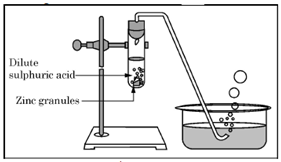1. Carbon di-oxide which extinguishes the burning candle.
2. Oxygen due to which the candle burns more brightly.
3. Sulphur dioxide which produces a suffocating smell.
4. Hydrogen which while burning produces a popping sound.

Question 4.

Sodium reacts with water to form sodium hydroxide and hydrogen gas. The balanced equation which represents the above reaction is;

1. Na(s) + 2H2O(l) → 2NaOH(aq) + 2H2(g)
2. 2Na(s) + 2H2O(l) → 2NaOH(aq) + H2(g)
3. 2Na(s) + 2H2O(l) → NaOH(aq) + 2H2(g)
4. 2Na(s) + H2O(l) → 2NaOH(aq) + 2H2(g)

Question 5.

Which of the options in the given table are correct?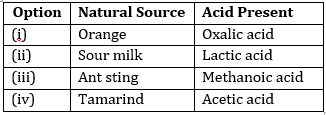1. (i) and (ii)
2. (i) and (iv)
3. (ii) and (iii)
4. (iii) and (iv)

Question 6.

C6H12O6(aq) + 6O2(aq) → 6CO2(aq) + 6H2O(l)

The above reaction is a/an

1. Displacement reaction
2. Endothermic reaction
3. Exothermic reaction
4. neutralisation reaction

Question 7.

Which of the following statements about the reaction given below are correct?

MnO2 + 4HCl → MnCl2 + 2H2O + Cl2

(i) HCl is oxidized to Cl2

(ii) MnO2 is reduced to MnCl

(iii) MnCl2 acts as an oxidizing agent

(iv) HCl acts as on oxidizing agent

1. (ii), (iii) and (iv)
2. (i), (ii) and (iii)
3. (i) and (ii) only
4. (iii) and (iv) only

Question 8.

Select from the following the statement which is true for bases.

1. Bases are bitter and turn blue litmus red.
2. Bases have a pH less than 7.
3. Bases are sour and change red litmus to blue.
4. Bases turn pink when a drop of phenolphthalein is added to them.

Question 9.

Study the following table and choose the correct option: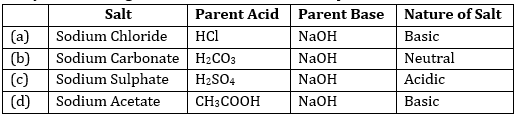Question 10.

It is important to balance the chemical equations to satisfy the law of conservation of mass. Which of the following statements of the law is incorrect?

1. The total mass of the elements present in the reactants is equal to the total mass of the elements presents in the products.
2. The number of atoms of each element remains the same, before and after a chemical reaction.
3. The chemical composition of the reactants is the same before and after the reaction.
4. Mass can neither be created nor can it be destroyed in a chemical reaction.

Question 11.

Consider the following statements in connection with the functions of the blood vessels marked A and B in the diagram of a human heart as shown.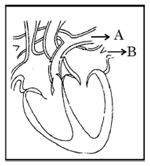(i) Blood vessel A - It carries carbon dioxide rich blood to the lungs.

(ii) Blood vessel B - It carries oxygen rich blood from the lungs.

(iii) Blood vessel B - Left atrium relaxes as it receives blood from this blood vessel

(iv) Blood vessel A - Right atrium has thick muscular wall as it has to pump blood to this blood vessel.

The correct statements are:

1. (i) and (ii) only
2. (ii) and (iii) only
3. (ii), (iii) and (iv)
4. (i),(ii) and (iii)

Question 12.

In living organisms during respiration which of the following products are not formed if oxygen is not available?

1. Carbon dioxide + Water
2. Carbon dioxide + Alcohol
3. Lactic acid + Alcohol
4. Carbon dioxide + Lactic Acid

Question 13.

The correct statements with reference to single celled organisms are

(i) Complex substances are not broken down into simpler substances.

(ii) Simple diffusion is sufficient to meet the requirement of exchange of gases.

(iii) Specialised tissues perform different functions in the organism.

(iv) Entire surface of the organism is in contact with the environment for taking in food.

1. (i) and (iii)
2. (ii) and (iii)
3. (ii) and (iv)
4. (i) and (iv)

Question 14.

Which one among the following is not removed as a waste product from the body of a plant?

1. Resins and Gums
2. Urea
3. Dry Leaves
4. Excess Water

Question 15.

Which of the following statements are correct in reference to the role of A (shown in the given diagram) during a breathing cycle in human beings?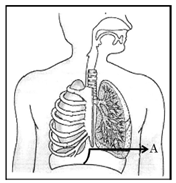(i) It helps to decrease the residual volume of air in lungs.

(ii) It flattens as we inhale.

(iii) It gets raised as we inhale.

(iv) It helps the chest cavity to become larger.

1. (ii) and (iv)
2. (iii) and (iv)
3. (i) and (ii)
4. (i), (ii) and (iv)

Question 16.

Which one of the following conditions is true for the state of stomata of a green leaf shown in the given diagram?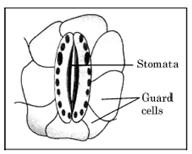1. Large amount of water flows into the guard cells.
2. Gaseous exchange is occurring in large amount.
3. Large amount of water flows out from the guard cells.
4. Large amount of sugar collects in the guard cells.

Question 17.

In which of the following is a concave mirror used?

1. A solar cooker
2. A rear view mirror in vehicles
3. A safety mirror in shopping malls
4. In viewing full size image of distant tall buildings.

Question 18.

A student wants to obtain magnified image of an object AB as on a screen. Which one of the following arrangements shows the correct position of AB for him/her to be successful?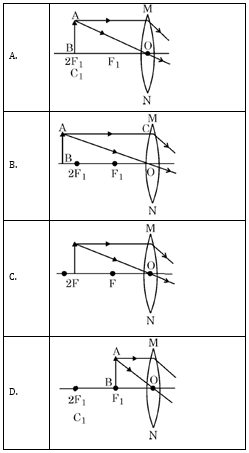Question 19.

The following diagram shows the use of an optical device to perform an experiment of light. As per the arrangement shown, the optical device is likely to be a;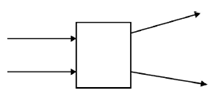1. Concave mirror
2. Concave lens
3. Convex mirror
4. Convex lens

Question 20.

A ray of light starting from air passes through medium A of refractive index 1.50, enters medium B of refractive index 1.33 and finally enters medium C of refractive index 2.42. If this ray emerges out in air from C, then for which of the following pairs of media the bending of light is least?

1. air-A
2. A-B
3. B-C
4. C-air

Question 21.

Which of the following statements is not true for scattering of light?

1. Colour of the scattered light depends on the size of particles of the atmosphere.
2. Red light is least scattered in the atmosphere.
3. Scattering of light takes place as various colours of white light travel with different speed in air.
4. The fine particles in the atmospheric air scatter the blue light more strongly than red. So the scattered blue light enters our eyes.

Question 22.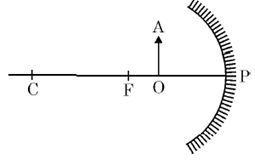For the diagram shown, according to the new Cartesian sign convention the magnification of the image formed will have the following specifications:

1. Sign — Positive, Value — Less than 1
2. Sign — Positive, Value — More than 1
3. Sign — Negative, Value — Less than 1
4. Sign — Negative, Value — More than 1

Question 23.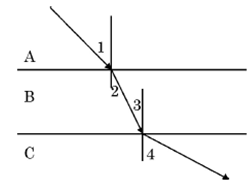A ray of light is incident as shown. If A, B and C are three different transparent media, then which among the following options is true for the given diagram?

1. ∠1 > ∠4
2. ∠1< ∠2
3. ∠3 = ∠2
4. ∠3 > ∠4

Question 24.

In the diagram given below. X and Y are the end colours of the spectrum of white light. The colour of 'Y' represents the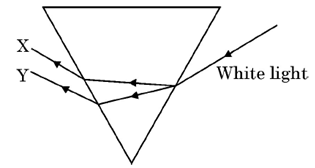1. Colour of sky as seen from earth during the day.
2. Colour of the sky as seen from the moon.
3. Colour used to paint the danger signals.
4. Colour of sun at the time of noon.

SECTION – B

Section-B consists of 24 questions (Q. No. 25 to 48). Attempt any 20 questions from this section.

The first attempted 20 questions would be evaluated.

Question 25.

Which one of the following reactions is categorised as thermal decomposition reaction?

1. 2H2O(Ɩ) —> 2H2(g) + O2(g)
2. 2AgBr(s) —> 2Ag(s) + Br2(g)
3. 2AgCƖ (s) —> 2Ag(s) + CƖ2(g)
4. CaCO3(s) —> CaO(s) + CO2(g)

Question 26.

Consider the pH value of the following acidic samples: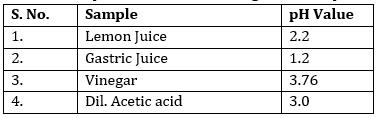The decreasing order of their H+ ion concentration is

1. 3 > 4 > 1 > 2
2. 2 > 1 > 3 > 4
3. 2 > 1 > 4 > 3
4. 3 > 4 > 2 > 1

Question 27.

Study the experimental set up shown in given figure and choose the correct option from the following: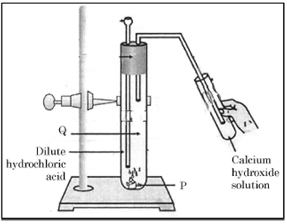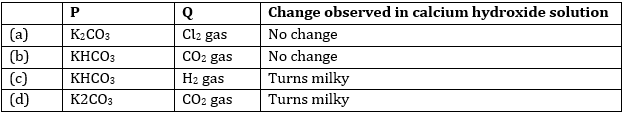Question 28.

Which one of the following structures correctly depicts the compound CaCl2?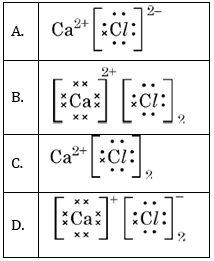Question 29.

The pair(s) which will show displacement reaction is/are

(i) NaCƖ solution and copper metal

(ii) AgNO3 solution and copper metal

(iii) Al,(SO4)3 solution and magnesium metal

(iv) ZnSO4 solution and iron metal

1. (ii) only
2. (ii) and (iii)
3. (iii) and (iv)
4. (i) and (ii)

Question 30.

Which of the following salts do not have the water of crystalisation?

(i) Bleaching Powder

(ii) Plaster of Paris

(iii) Washing soda

(iv) Baking soda

1. (ii) and (iv)
2. (i) and (iii)
3. (ii) and (iii)
4. (i) and (iv)

Question No. 31 to 35 consist of two statements – Assertion (A) and Reason (R).

Answer these questions selecting the appropriate option given below:

1. Both A and R are true and R is the correct explanation of A
2. Both A and R are true and R is not the correct explanation of A
3. A is true but R is false
4. A is False but R is true

Question 31.

Assertion (A): Sodium hydrogen carbonate is used as an ingredient in antacids.

Reason (R): NaHCO3 is a mild non-corrosive basic salt.

Question 32.

Assertion (A): Burning of Natural gas is an endothermic process.

Reason (R): Methane gas combines with oxygen to produce carbon dioxide and water.

Question 33.

Assertion (A): Nitrogen is an essential element for plant growth and is taken up by plants in the form of inorganic nitrates or nitrites.

Reason (R): The soil is the nearest and richest source of raw materials like Nitrogen. Phosphorus and other minerals for the plants.

Question 34.

Assertion (A): Sun appears reddish at the time of Sunrise and Sunset.

Reason (R): Distance travelled by sunlight in the atmosphere is lesser during sunrise and sunset as compared to noon.

Question 35.

Assertion (A): Hydrochloric acid helps in the digestion of food in the stomach.

Reason (R): Hydrochloric acid creates an acidic medium to activate protein digesting enzymes.

Question 36.

A student was asked to write a stepwise procedure to demonstrate that carbon dioxide is necessary for photosynthesis. He wrote the following steps. The wrongly worded step is –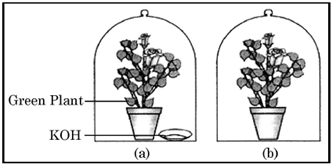1. Both potted plants are kept in dark room for at least three days.
2. Bottom of the bell jars is sealed to make them air tight.
3. Both potted plants are kept in sunlight after the starch test.
4. A leaf from both the plants is taken to test the presence of starch.

Question 37.

Respiratory structures of two different animals-a fish and a human being are as shown.

Observe (a) and (b) and select one characteristic that holds true for both of them.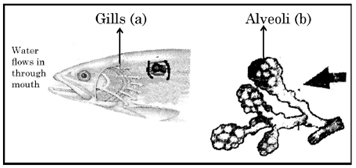1. Both are placed internally in the body of animal.
2. Both have thin and moist surface for gaseous exchange.
3. Both are poorly supplied with blood vessels to conserve energy.
4. In both the blood returns to the heart after being oxygenated.

Question 38.

Observe the diagram of an activity given below. What does it help to conclude, when the person exhales into the test-tube?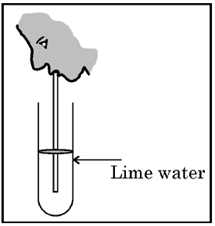1. Percentage of carbon dioxide is more in inhaled air.
2. Fermentation occurs in the presence of oxygen.
3. Percentage of carbon dioxide is more in the exhaled air.
4. Fermentation occurs in the presence of carbon dioxide.

Question 39.

If a lens can converge the sun rays at a point 20 cm. away from its optical centre, the power of this lens is –

1. + 2D
2. - 2D
3. + 5D
4. - 5D

Question 40.

The radius of curvature of a converging mirror is 30 cm. At what distance from the mirror should an object be placed so as to obtain a virtual image?

1. Infinity
2. 30 cm
3. Between 15 cm and 30 cm
4. Between 0 cm and 15 cm

Question 41.

The length of small intestine in a deer is more as compared to the length of small intestine of a tiger. The reason for this is -

1. Mode of intake of food.
2. Type of food consumed.
3. Presence or absence of villi in intestines.
4. Presence or absence of digestive enzymes.

Question 42.

Identify the two components of Phloem tissue that help in transportation of food in plants.

1. Phloem parenchyma & sieve tubes
2. Sieve tubes & companion cells
3. Phloem parenchyma & companion cells
4. Phloem fibres and sieve tubes

Question 43.  .

A converging lens forms a three times magnified image of an object, which can be take on a screen. If the focal length of the lens is 30 cm, then the distance of the object from the lens is

1. 55 cm
2. – 50 cm
3. – 45 cm
4. – 40 cm

Question 44.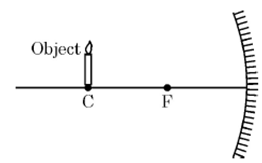Which of the following statements is not true in reference to the diagram shown above?

1. Image formed is real.
2. Image formed is enlarged.
3. Image is formed at a distance equal to double the focal length.
4. Image formed is inverted.

Question 45.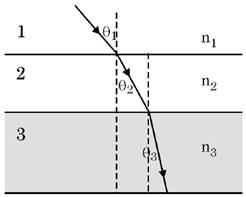In the diagram shown above n1, n2 and n3 are refractive indices of the media 1, 2 and 3 respectively which one of the following is true in this case?

1. n1 = n
2. n1 > n2
3. n2 > n3
4. n3 >  n1

Question 46.

The refractive index of medium A is 1.5 and that of medium B is 1.33. If the speed of light in air is 3 ⨯ 108 m/s, what is the speed of light in medium A and B respectively?

1. 2 × 108 m/s and 1.33 × 108 m/s
2. 1.33 × 108 m/s and 2 × 108 m/s
3. 2.25 × 108 m/s and 2 × 108 m/s
4. 2 × 108 m/s and 2.25 × 108 m/s

Question 47.

An object of height 4 cm is kept at a distance of 30 cm from the pole of a diverging mirror. If the focal length of the mirror is 10 cm, the height of the image formed is

1. + 3.0 cm
2. + 2.5 cm
3. + 1.0 cm
4. + 0.75 cm

Question 48.

50.0 mL of tap water was taken in a beaker. Hydrochloric acid was added drop by drop to water. The temperature and pH of the solution was noted. The following graph was obtained. Choose the correct statements related to this activity.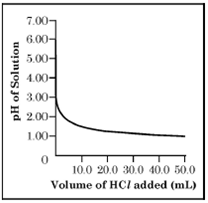(i) The process of dissolving an acid in water is highly endothermic.

(ii) The pH of the solution increases rapidly on addition of acid.

(iii) The pH of the solution decreases rapidly on addition of acid.

(iv) The pH of tap water was around 7.0.

1. (i) and (ii)
2. (i) and (iii)
3. (iii) and (iv)
4. (ii) and (iv)

SECTION – C

Section-C consists of three cases followed by questions. There are a total of 12 questions (Q. No. 49 to 60) in this section. Attempt any 10 questions from this section. The first attempted 10 questions would be evaluated.

Case-I:

A student, took four metals P, Q, R and S and carried out different experiments to study the properties of metals. Some of the observations were:

• All metals could not be cut with knife except metal R.
• Metal P combined with oxygen to form an oxide M2O3 which reacted with both acids and bases.
• Reaction with water.

P - Did not react either with cold or hot water but reacted with steam

Q - Reacted with hot water and the metal started floating

R - Reacted violently with cold water.

S - Did not react with water at all

Based on the above observations answer the following:

Question 49.

Out of the given metals, the one which needs to be stored used Kerosene is

Question 50.

Out of the given metals, the metal Q is

1. Iron
2. Zinc
3. Potassium
4. Magnesium

Question 51.

Metal which forms amphoteric oxides is

Question 52.

The increasing order of the reactivity of the four metals is;

1. P < Q < R < S
2. S < R < Q < P
3. S < P < Q < R
4. P < R < Q < S

Case-II:

The figure shown below represents a common type of dialysis called as Hemodialysis. It removes waste products from the blood. Such as excess salts, and urea which are insufficiently removed by the kidney in patients with kidney failure. During the procedure, the patient's blood is cleaned by filtration through a series of semi-permeable membranes before being returned to the blood of the patient. On the basis of this, answer the following questions: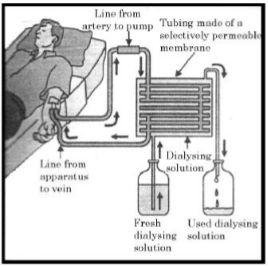Question 53.

The haemodialyzer has semi-permeable lining of tubes which help to:

1. To maintain osmotic pressure of blood.
2. To filter nitrogenous wastes from the dialyzing solution.
3. In passing the waste products in the dialyzing solution.
4. To pump purified blood back into the body of the patient.

Question 54.

Which one of the following is not a function of Artificial Kidney?

1. To remove nitrogenous wastes from the blood.
2. To remove excess fluids from the blood.
3. To reabsorb essential nutrients from the blood.
4. To filter and purify the blood.

Question 55.

The fused dialysing' solution is rich in;

1. Urea and excess salts
2. Blood cells
3. Lymph
4. Proteins

Question 56.

Which part of the nephron in human kidney, serves the function of reabsorption of certain substances?

1. Glomerulus
2. Bowmans Capsule
3. Tubules
4. Collecting duct

Case-III:

A compound microscope is an instrument which consists of two lenses L1 and L2. The lens L1 called objective, forms a real, inverted and magnified image of the given object. This serves as the object for the second lens L2; the eye piece. The eye piece functions like a simple microscope or magnifier. It produces the final image, which is inverted with respect to the original object, enlarged and virtual.

Question 57.

What types of lenses must be L1 and L2

1. Both concave
2. Both convex
3. L1 - concave and L2 - convex
4. L- convex and L2 - concave

Question 58.

What is the value and sign of magnification (according to the new Cartesian sign convention) of the image formed by L1

1. Value = Less than 1 and Sign = Positive
2. Value = More than 1 and Sign = Positive
3. Value = Less than 1 and Sign = Negative
4. Value = More than 1 and Sign = Negative

Question 59.

What is the value and sign of (according to new Cartesian sign convention) magnification of the image formed by L2?

1. Value = Less than 1 and Sign = Positive
2. Value = More than 1 and Sign = Positive
3. Value = Less than 1 and Sign = Negative
4. Value = More than 1 and Sign = Negative

Question 60.

If power of the eyepiece (L2) is 5 diopters and it forms an image at a distance of 80 cm from its optical centre, at what distance should the object be?

1. 12 cm
2. 16 cm
3. 18 cm
4. 20 cm

Ans 1.

Correct option – C: White, BaSO

When an aqueous solution of sodium sulphate reacts with an aqueous solution of barium chloride, insoluble white coloured barium sulphate along with solution of sodium chloride is formed.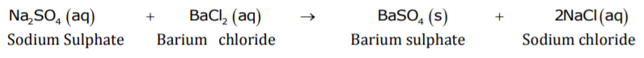Ans 2.

Correct option – A: (i) and (iii)

Na2O and K2O are soluble in water to form alkalis

Na2O + H2O → 2NaOH           K2O + H2O → 2KOH

Ans 3.

Correct option – D: Hydrogen which while burning produces a popping sound.

When a metal reacts with acid then hydrogen gas is evolved. When a burning stick is brought near the mouth of the test tube then the evolving hydrogen gas is burnt with a pop sound.

Ans 4.

Correct option – B: 2Na(s) + 2H2O(l) → 2NaOH(aq) +H2(g)

Ans 5.

Correct option – C: (ii) and (iii)

Sour milk consist of lactic acid and ant sting has methanoic acid.

Ans 6.

Correct option – C: Exothermic reaction

During respiration, carbohydrates (glucose) combine with oxygen in the cell and energy is released hence it is considered an exothermic reaction.

C6H12O6 +6O2 → 6CO2 + 6H2O + Energy

Ans 7.

Correct option – C: (i) and (ii) only

HCl is oxidized to Cl2 and MnO2 is reduced to MnCl2.

Ans 8.

Correct option – D

Bases turn pink when a drop of phenolphthalein is added to them.

Ans 9.

Correct option – D: Sodium Acetate – CH3COOH – NaOH – Basic.

Sodium acetate is a basic salt derived from a strong base NaOH and a weak acid CH3COOH.

Ans 10.

Correct option – D: Mass cannot be created nor destroyed.

The law of conservation of mass states that mass can neither be created nor destroyed in a chemical reaction.

Ans 11.

Correct option – A: (i) and (ii) only

Blood vessel A is pulmonary artery while blood vessel B is pulmonary vein. The pulmonary arteries carry low-oxygen blood from the right ventricle of the heart to the lungs. The pulmonary veins carry oxygenated blood from the lungs to the left atrium of the heart.

Ans 12.

Correct option – C: Lactic acid + Alcohol

Alcohol is produced during fermentation in yeast while lactic acid is produced during anaerobic respiration in human muscle cells.

Ans 13.

Correct option – C: (ii) and (iv)

In unicellular organisms, simple diffusion is sufficient to carry out the process of gaseous exchange. They do not need specific organs for taking in food, exchange of gases or removal of wastes because the entire surface of the organism is in contact with the environment.

Ans 14.

Correct option – B: Urea

Plants produce release oxygen and carbon dioxide, excess water, gums, oils, latex and resins as waste products. Urea is not produced in plants.

Ans 15.

Correct option – D: (i), (ii) and (iv)

A is diaphragm which flattens as we inhale air, helps to decrease the residual volume of air in lungs and helps the chest cavity to become larger.

Ans 16.

Correct option – C: Large amount of water flows out from the guard cells.

A large amount of water flows out from the guard cells thereby closing the stomata.

Ans 17.

Correct option – A: A solar cooker

A concave mirror is used in a solar cooker.

Ans 18.

Correct option – C.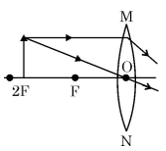In order to obtain the magnified image on the screen the object must be placed between F and 2F of convex lens. In fourth option, the image obtained will be magnified but it cannot be obtained on the screen as it will be formed at infinity. So correct option is option c).

Ans 19.

Correct option – B: concave lens

In given diagram, we can see that the light rays after refraction are getting diverged. Thus, the optical device used in the arrangement is concave lens.

Ans 20.

Correct option – B: A-B

In case of medium A and medium B, the difference between their refractive indices is less than any other paper. Thus, the bending of the light will be least for these two media. So correct option is b).

Ans 21.

Correct option – C: Scattering of light takes place as various colours of white light travel with different speed in air.

All other statements except option c) are correct. So correct option will be untrue statement which is option c).

Ans 22.

Correct option – B:  sign – positive, value more than 1

In the diagram given, the optical device used is concave mirror and object is placed between focus and pole. When object is placed between focus and pole of concave mirror the image formed is virtual, erect and magnified. As the image will be virtual and erect according to the sign convention the image formed will have magnification with positive sign and as it is magnified the value of magnification will be more than 1.

Ans 23.

Correct option – C:   ∠3 = ∠2

By observing the image it is clear that, only relation possible from the given option is option c).

Ans 24.

Correct option – C: Colour used to paint the danger signals.

It is given that X and Y are the end colours of spectrum. The end colours of the spectrum are violet and red. The prism is placed inverted but X ray has bent more towards the base and Y is least bent. Thus, Y represent red and X represents violet colour. Red colour is used to paint danger signals. Thus, correct option is c).

SECTION - B

Ans 25.

Correct option – D: CaCO3 ⟶ CaO + CO2

Reactions in which a substance is dissociated into two or more than two substances by applying heat is thermal decomposition reaction. CaCO3 requires heat to convert into CaO and CO2.

Ans 26.

Correct option – C: 2 > 1 > 4 > 3

pH = -log[H+]

When pH decreases then H+ ion concentration increases.

Ans 27.

Correct option – D: P = K2CO3, Q = CO2 gas, Turns milky

When acid reacts with metal carbonate it releases CO2 which turns solution milky.

Ans 28.

Correct option – D.

After donating 2 electrons, Ca is having 8 electrons in outermost shell and after donating 1 electron to 1 Cl atoms, Cl atom also have 8 electrons in outermost shell and becomes stable.

Ans 29.

Correct option – C: (iii) and (iv)

Metals who are above have higher reactivity than metals who are below in reactivity series. Metals with higher reactivity displaces the metals with low reactivity.

Ans 30.

Correct option – D: (i) and (iv)

Bleaching powder- Ca(OCl)2

Baking Soda- NaHCO3

Washing Soda- Na2CO3.10H2O

Plaster of Paris- CaSO4.1/2H2O

Ans 31.

Correct option – A: Both (A) and (R) are true and (R) is the correct explanation of (A).

Sodium bicarbonate is non corrosive basic salt so it is used as antacid to treat acidity.

Ans 32.

Correct option – D: (A) is false, but (R) is true.

When methane (natural gas) reacts with oxygen, the result is carbon di-oxide and water, along with heat, hence making it an exothermic reaction.

Ans 33.

Correct option – B: Both (A) and (R) are true but (R) is not the correct explanation of (A).

Nitrogen is an essential element for plant growth and is taken up by plants in the form of inorganic nitrates or nitrites. The soil is the nearest and richest source of nitrogen, phosphorus and other minerals for the plants.

Ans 34.

Correct option – C: (A) is true, but (R) is false.

Sun appears reddish at sunrise and sunset is correct. But during sunrise and sunset the sun is at horizon and it has to travel more distance during this time. Thus, (R) statement is false statement.

Ans 35.

Correct option – A: Both (A) and (R) are true and (R) is the correct explanation of (A).

Hydrochloric acid creates an acidic medium to activate protein digesting enzymes. Thus, it helps in the digestion of food in the stomach.

Ans 36.

Correct option – C: Both potted plants are kept in sunlight after the starch test.

Plants get destarched when kept in dark because in dark they are not able to produce food by the process of photosynthesis as there is no sunlight available to run this process.

Ans 37.

Correct option – B: Both have thin and moist surface for gaseous exchange.

Both the respiratory structures, gills and alveoli have thin and moist surface for gaseous exchange. They are richly with blood vessels to conserve energy.

Ans 38.

Correct option – C: Percentage of carbon dioxide is more in the exhaled air.

When we exhale air into a test-tube containing lime water, the water turns milky. This shows that exhaled air contains more carbon dioxide than inhaled air.

Ans 39.

Correct option – C: +5D

It is said that the sun rays are converged at 20 cm from optical centre. This means lens is convex lens and have focal length 20 cm.

f = 20 cm = 0.2 m

Power of lens, P = 1/f (in metre) = 1/ 0.2 = +5D

Ans 40.

Correct option – D: Between 0 cm and 15 cm

The mirror is concave mirror (converging mirror). The radius of curvature is 30 cm which means the focal length will be 15 cm. For concave mirror, to obtain the virtual image the object must be placed between pole and focus. Thus, the object must be placed between 0 cm and 15 cm.

Ans 41.

Correct option – B: Type of food consumed.

Herbivores mainly eat plants. Plants contain cellulose, which is difficult to digest. Hence, they have the longest small intestine.

Ans 42.

Correct option – B: Sieve tubes and companion cells

The food (sugar) made in leaves is loaded into the sieve tubes of phloem tissue by using the energy derived from ATP. The companion cells make use of the transmembrane proteins for the uptake of sugar and amino acids by active transport.

Ans 43.

Correct option – D: -40 cm

Given that,

Size of image, h’ = 3 × size of object, hFocal length, f = 30 cm

For the given setup converging lens or convex lens is used and magnification of the convex lens is expressed asv=-3u

Here, the object distance is considered as negative since it will be placed towards the negative x-axis, whereas both focus and image will be measured toward the right side of the lens (i.e., +x-axis) as we can see below.

Now the relation between image distance (v), object distance (u) and focal length (f) can be expressed as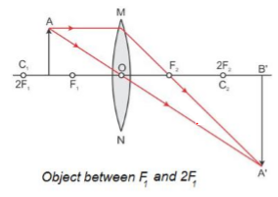Ans 44.

Correct option – C: Image is formed at a distance equal to double the focal length.

The ray diagram for the given case can be illustrated as shown below and from this, we conclude that if the size of the image and object are equal and the object and image distance will also be the same when the object is placed at the centre of curvature (i.e., C = 2f).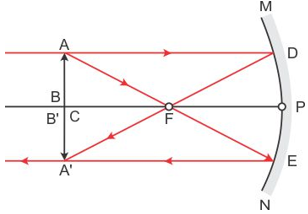Ans 45.

Correct option – D: n3 > n1

From the given diagram we can see that when the light ray enters from 1st to 2nd medium it bends towards normal which means the refractive index of the second medium is greater than the first medium (i.e., n1 < n2), similarly we can conclude that n2 < n3.

Hence for the given case, the correct option is d, since n3 > n1.

Ans 46.

Correct option – D: 2 × 108 m/s and 2.25× 108 m/s

Given that,

Refractive index of medium A, nA = 1.5

Refractive index of medium B, nB = 1.33

Speed of light in air, c = 3 × 108 m/s

Now the refractive index of medium A with respect to air can be given asAns 47.

Correct option – C: +1 cm

Object height, h = 4 cm

Object distance, u = -30 cm

Focal length, f =10 cm

Now as we know the relation between focal length, image distance and object distance can be predicted using the mirror formula as shown below.Ans 48.

Correct option – C: (iii) and (iv)

The pH of the solution decreases rapidly on addition of acid. And the pH of tap water was around 7.0.

SECTION – C

Ans 49.

Correct option – B:  R

R reacts violently with water so it is stored in kerosene.

Ans 50.

Correct option – D:  Magnesium

Magnesium starts floating due to the bubbles of hydrogen gas sticking to its surface. As the Bubbles move up it pulls Mg with it.

Ans 51.

Correct option – A:  P

Al, Zn and Fe does not react with cold or hot water but reacts with steam. Al forms Al2O3. Al is amphoteric in nature (P=Al).

Ans 52.

Correct option – C:  S < P < Q

P = Al, Q = Mg, R = Na or K, S = Cu/Ag/Au/Pt

According to metal reactivity series   R > Q > P > S

Ans 53.

Correct option – C:  In passing the waste products in the dialyzing solution.

The haemodialyzer has semi-permeable lining of tubes which help in passing the waste products in the dialyzing solution.

Ans 54.

Correct option – A: To reabsorb essential nutrients from the blood.

Unlike natural kidneys, artificial kidneys are unable to absorb essential nutrients which might have passed into the blood during the filtration process.

Ans 55.

Correct option – A: Urea and excess salts.

The 'used dialysing' solution is rich in urea and excess salts such as sodium, potassium, calcium, chloride, magnesium and bicarbonates.

Ans 56.

Correct option – C: Tubules

The proximal and distal tubules, the loop of Henle, and the collecting ducts are sites for the reabsorption of water and ions.

Ans 57.

Correct option – A: Both convex

As we know for concave lens image formed is always virtual, erect and diminish hence in our case according to the given condition both lens L¬1 and L2¬ are convex lenses since both produce enlarged and inverted images.

Ans 58.

Correct option – D: Value = More than 1 and Sign = Negative.

For the given case the image is inverted and magnified for L1.

Hence the value of the magnification is more than 1 since the height of the image is greater than the object and it will be negative to show the image is inverted.

i.e.,M=-h^'/h…(here,h1)Ans 59.

Correct option – D: Value = More than 1 and Sign = Negative.

For the given case the image is inverted and magnified for L2.

Hence the value of the magnification is more than 1 since the height of the image is greater than the object and it will be positive to show the image is erect and virtual.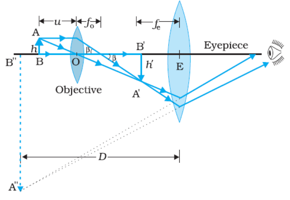Note: In the given case image formed by lens L1 is considered as an object hence image formed by L2 will be erect and virtual as we can see above.Ans 60.

Correct option –B: 16 cm

Given,

Power of eyepiece of lens 2, P2 = 5 D

i.e., f = 1/5 = 0.2 m = 20 cm

Image distance, v =- 80 cm

Note: Here image distance is considered as negative since the image formed by L2 is virtual and it will be formed along the negative x-axis or behind the lens.

Now by using the lens formula we know thatu=-16 cm

## Why to choose our CBSE Class 10 Science Study Materials?

• Contain 950+ video lessons, 200+ revision notes, 8500+ questions and 15+ sample papers
• Based on the latest CBSE syllabus
• Free textbook solutions & doubt-solving sessions
• Ideal for quick revision
• Help score more marks in the examination
• Increase paper-solving speed and confidence with weekly tests

### STUDY RESOURCES

REGISTERED OFFICE : First Floor, Empire Complex, 414 Senapati Bapat Marg, Lower Parel, Mumbai - 400013, Maharashtra India.